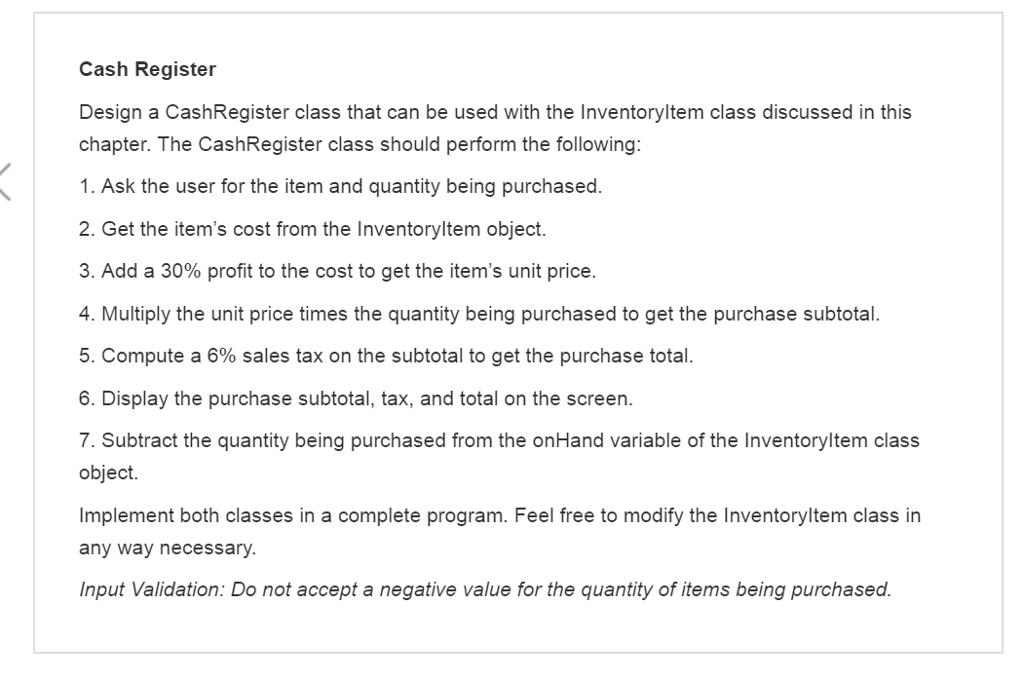# Question & Answer: Cash Register Design a CashRegister class that can be used with the InventoryItem class discussed in th…..Cash Register Design a CashRegister class that can be used with the InventoryItem class discussed in this chapter. The CashRegister class should perform the following: 1. Ask the user for the item and quantity being purchased. 2. Get the item’s cost from the InventoryItem object. 3. Add a 30% profit to the cost to get the item’s unit price. 4. Multiply the unit price times the quantity being purchased to get the purchase subtotal. 5. Compute a 6% sales tax on the subtotal to get the purchase total. 6. Display the purchase subtotal, tax, and total on the screen. 7. Subtract the quantity being purchased from the onHand variable of the InventoryItem class object. Implement both classes in a complete program. Feel free to modify the InventoryItem class in any way necessary. Input Validation: Do not accept a negative value for the quantity of items being purchased.

Don't use plagiarized sources. Get Your Custom Essay on
Question & Answer: Cash Register Design a CashRegister class that can be used with the InventoryItem class discussed in th…..
GET AN ESSAY WRITTEN FOR YOU FROM AS LOW AS \$13/PAGE

Here is the coding section as per the given scenerio, please go through it thoroughly;-

//This program demonstrates a class with a destructor
#include <iostream>
#include <iomanip>
#include “price.h”
#include “Sale.h”
#include “Inventory_Item.h”
using namespace std;
int main()
{
int num_Selected;
double quantity_Selected;
char again;
double price;
double cost;
const int NUM_ITEMS = 5;
Inventory_Item inventory[] = {
Inventory_Item(“Hardboard”, 6.95, 12),
Inventory_Item(“Wood”, 8.75, 20),
Inventory_Item(“Plies”, 3.75, 10),
Inventory_Item(“Wooden_boxes”, 7.95, 14),
Inventory_Item(“Card_board”, 2.50, 22) };
do
{
cout << “Here is a list of our current inventory” << endl;
cout << ” ” << endl;

cout << setw(7) << “Item Number” <<
cout << setw(14) << “Description”
<< setw(8) << “Cost” << setw(8)
<<setw(20) << “Units on Handn”;
cout << “==========================================================n”;
for (int i = 0; i < NUM_ITEMS; i++)
{
cout << setw(7) << i;
cout << setw(21) << inventory[i].getDescription();
cout << setw(14) << inventory[i].getCost();
if (quantity_Selected == 0)
cout << setw(7) << inventory[i].getUnits() << endl;
else
cout << setw(7) << inventory[i].getUnits() – quantity_Selected << endl;
}
cout << “” << endl;
cout << “” << endl;
cout << “Please enter the item number you wish to purchase: “;
cin >> num_Selected;
while((num_Selected < 0) || (num_Selected > 5))
{
cout << “Please enter an inventory item number 0-5: “;
cin >> num_Selected;
}
cout << “Please enter the quantity you wish to purchase: “;
cin >> quantity_Selected;
while(quantity_Selected > inventory[num_Selected].getUnits())
{
cout << “Please enter quantity less than or equal to ” << inventory[num_Selected].getUnits() << ” units: “;
cin >> quantity_Selected;
}

price = inventory[num_Selected].getCost();

cout << ” ” << endl;
cout << “Here is the bill: ” << endl;
cout << ” ” << endl;
Sale2 itemSale2(price);
double subTotal = itemSale2.getTotalUnitPrice() * quantity_Selected;

cout << “Subtotal:t \$” << subTotal << endl;
cost = subTotal;
Sale itemSale(cost);

cout << “Sales tax:t \$” << itemSale.getTax() << endl;
cout << “Total:tt \$” << itemSale.getTotal() << endl;
cout << “Do you want to make another purchase? “;
cin >> again;
cout << ” ” << endl;
}
while (again == ‘Y’ || again == ‘y’);
return 0;
}